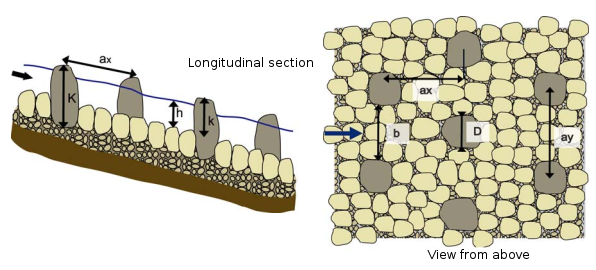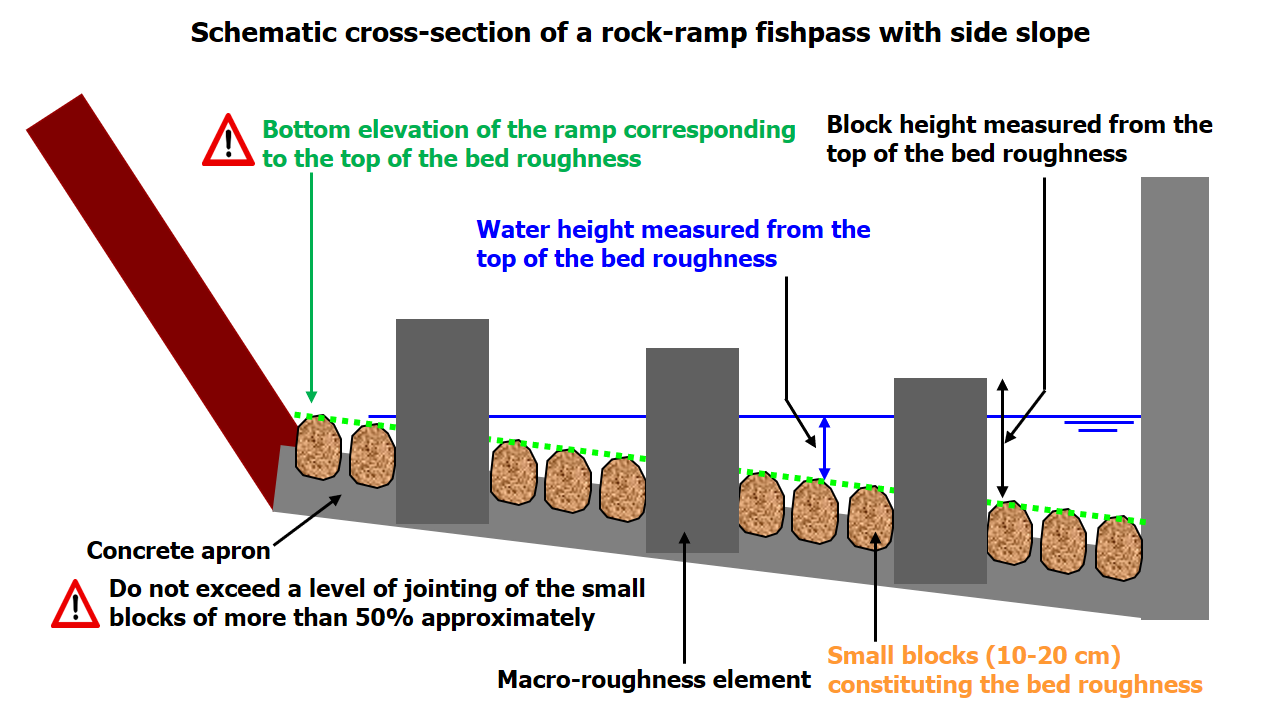# Rock-ramp fishpasses

The rock-ramp fishpass calculation module makes it possible to calculate the characteristics of a rock-ramp pass made up of uniformly distributed blocks with equal transverse $$ax$$ and longitudinal $$ay$$ spacings.Excerpt from Larinier et al., 20061

The tool allows you to calculate one of the following values:

• The width of the pass (m);
• The slope of the pass (m);
• The flow rate (m3/s);
• The depth $$h$$ (m);
• The concentration of the blocks.

It requires the following values to be entered:

• The upstream bottom coordinate (m);
• The length of the pass (m);
• The background roughness (m);
• The width of the blocks $$D$$ facing the flow (m);
• The useful height of the blocks $$k$$ (m);
• The drag coefficient of a single block (1 for round, 2 for square).

# Bed roughness1. Larinier, Michel, Courret, D., Gomes, P., 2006. Technical guide for the design of "natural" fish passes, GHAPPE RA Report. Compagnie Nationale du Rhône / Adour Garonne Water Agency. http://dx.doi.org/10.13140/RG.2.1.1834.8562